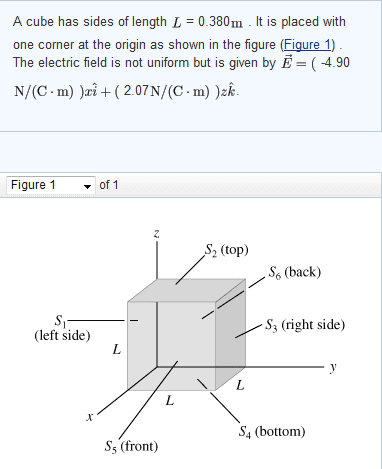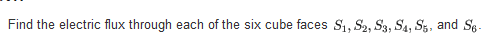# The Electric Flux on all sides of a cube

ecsx00

## Homework Statement## Homework Equations

$\phi$ = $\vec{E}$ * A * d
phi is the electric flux
E is the Electric field from vector form
A is the area and A = $\pi$$r^{2}$
d is the distance on the axis (x, y, or z)

## The Attempt at a Solution

s1 = s3 = 0 *Since they are along the y-axis and there is no y-vector
s2 = 1.14e-1
s4 = - 1.14e-1
s5 = 2.69e-1
s6 = -2.69e-1

I don't know why I am getting these wrong but you need to arrange them from s1-s6 on the answer when you submit them and it tells me to check my sign on s5. Changing it to negative doesn't help.
I'll recheck my calculations again and try a different method. I probably forgot to put " $\pi$" into the calculations but I will check on that.

Am I doing this right? Or is the equation to solve this problem totally different and I am off.
Pointing out where I went wrong helps, please do not post the answer as I just need a hint or tip.
Thank you!

Bhumble
Area for a square is the length times width not $\pi r^2$ .
You need to consider that flux is dependent on angle and that not all the surfaces are perpendicular to the electric field.
Also what is the deal with the distance component in you're equation for flux?

Homework Helper
I'd add as a hint: sketch the field.

ecsx00
Area for a square is the length times width not $\pi r^2$ .
You need to consider that flux is dependent on angle and that not all the surfaces are perpendicular to the electric field.
Also what is the deal with the distance component in you're equation for flux?

I really have no clue. This equation is from the book directly.

ecsx00
$\varphi$ = $\vec{E}$$L^{2}$cos(θ)
or
$\varphi$ = -$\vec{E}$$L^{2}$cos(θ)
Where E is the electric field from the $\hat{n}$ vector.
θ is either 90° or 180°

Is that the equation I am looking for?

Last edited:
Homework Helper
Instead of trying to guess which equation to apply - why don't you start with what "flux" actually means? And seriously: sketch the field.

Bhumble
Were you able to figure it out?
That equation looks right. Like Simon said though you should make sure to understand what flux means and you'll know when to apply the appropriate equations.

Dens
Use $$\oint \vec{E} \cdot \vec{dA}$$ What is E and dA? What is the value of the electric field along S5?

ecsx00
Thanks. I solved it after looking at a few examples that people had on this site and eventually figured out how they got to the solution. I wouldn't guess it.